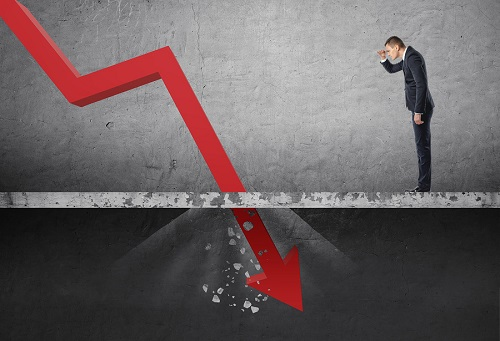# Accounting – What do you need to know about DepreciationMachinery, furniture, outdoor lighting, equipment, buildings, computers, vehicles, and parking lots are some of the assets that are likely last for more than one year but can’t last indefinitely. In every accounting period, a particular portion of such assets gets used up, perhaps in tear and wear and it is known as depreciation. You should report the depreciation expense in the income statement.

How to calculate depreciation

The calculation and reporting of depreciation expense are based on the following accounting principles:

Matching principle: According to this principle, you should allocate the cost of the asset to depreciation expense over the asset’s life. This implies that you should divide up the cost of the property and report the appropriate portion of this cost in each income statement issued during the asset’s life. In this case, you will be matching a fraction of the cost of asset with each period in which the asset was in use. Besides, the cost of asset is being matched with the revenue that the asset brought in during each accounting period.

Cost principle: According to this principle, you should report depreciation on the income statement while the asset should appear on the balance sheet as a contra account. The amounts reported on the financial statements must be based on the historical cost of the asset. You should never base the amount of the asset and depreciation expense on the current market value or the cost of replacing the asset.

Various methods can help you calculate depreciation in agreement with the cost principle and the matching principle. These methods can be grouped into the accelerated depreciation method and the straight-line depreciation method. The straight-line depreciation is widely used due to its simplicity. To calculate the depreciation using the straight-line method, charge an equal amount of the cost of the asset to every accounting period using the formula below.

Depreciation expense = (Asset’s historical cost – the salvage value) ÷ the asset’s useful life.

On the other hand, accelerated depreciation method provides a higher depreciation value in the early years and assigns less amount of depreciation in the later years of the asset’s useful life. Since depreciation is an expense, the company is likely to report fewer profits. Most businesses don’t desire this, and therefore, they opt for the straight-line depreciation method.

Conclusion

Most fixed assets depreciate over time. For effective financial reporting, you must recognize depreciation in your accounting books using accounting journal entries. If you aren’t sure of how to calculate and record depreciation in your business books, engage an accounting service in Singapore.